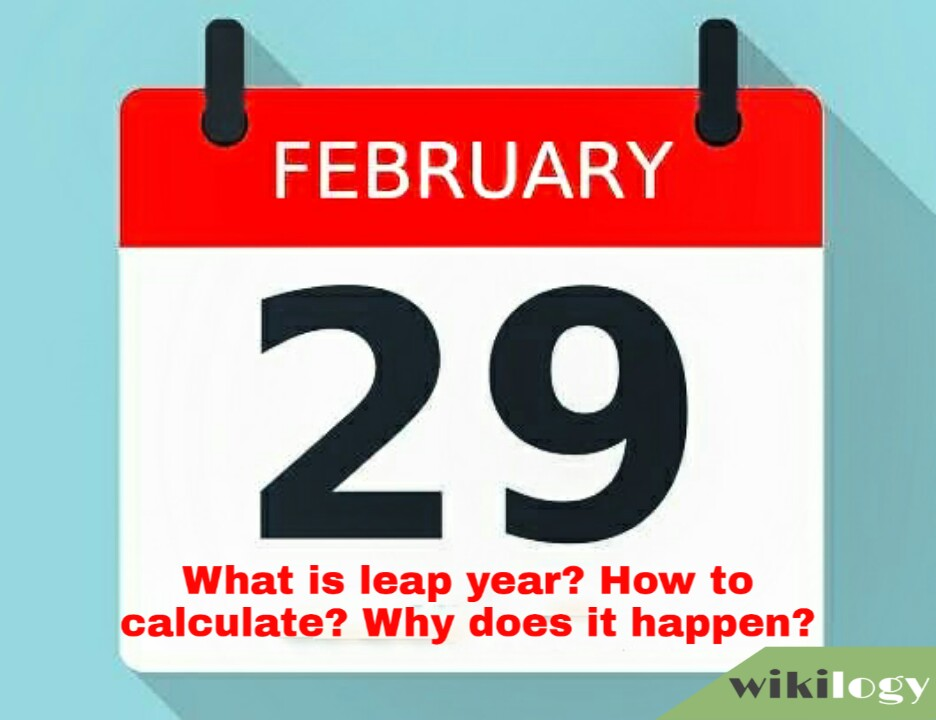# What is Leap Year? How to Calculate & Why does it Happen?What is Leap Year? How to Calculate & Why does it Happen?

Page Summary

Today, I will discuss what is leap year, how to calculate leap year and why leap year happens.

## What is a leap year?

The year which contains an extra day (366 days instead of 365 days) is known as a leap year. The day is added in February and it is 29th February (as per Gregorian Calendar). That’s why 29th February is called the leap day.

## Why does leap year happen?

Earth completes a single rotation around the sun exactly by 365.242375 days.
But we count 365 days in a year. So there have an extra 0.242375 days in a complete rotation. After 400 years there are about 96.95 or 97 days extra. To catch up these extra days we count a year of 366 days in every 4th year. If we count an extra day in every 4th year, we can get 100 (400/4) leap years in 400 years. That means 100 extra days are added in 400 years to catch up with the extra 97 days. But this is not also accurate as there are added extra 3 days. To resolve this problem we count 97 leap years in 400 years instead of 100 leap years. So we exclude 100th, 200th and 300th years (which are exactly divided by 100) from the list of leap years.

Nice to know that this rule was first applied by the Gregorian Calendar in 1582.

## How to calculate leap year?

To calculate a year whether it leap or not, you have to go through 3 simple steps.

• If the year is not divisible by 4, then it is not a leap year. If the year is divisible by 4, then go to the next step.
• If the year is not divisible by 100, then it is a leap year. If divisible, then go to the last step.
• If the year is divisible by 400, then it is a leap year. If not, then it is not a leap year.

By these three steps, we can find out if a year is a leap or not.

Example: Let’s test the year 2300.

• 1st step: 2300/4=575. As it is divisible by 4. So we have to go to the next step.
• 2nd step: 2300/100=23 and the remainder is 0. That means it is divisible by 100. So, let’s go to the last step.
• 3rd step: 2300/400=5 and reminder is 300. That means it is not divisible by 400. Thus it is confirmed that the year 2300 is not a leap year.

At the end of the article, my sincere sympathies with those, whose birthday is on 29th February. Don’t be sad. Let’s know your birthday by dropping a comment.

1.ESHABA